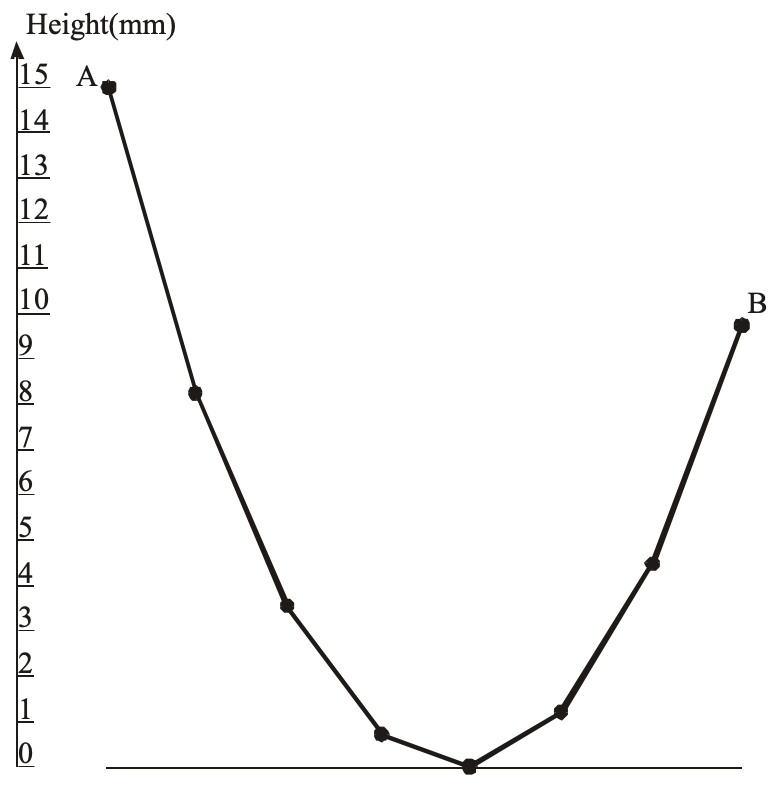시간 제한메모리 제한제출정답맞힌 사람정답 비율
1 초 128 MB0000.000%

## 문제The New Year garland consists of N lamps attached to a common wire that hangs down on the ends to which outermost lamps are affixed. The wire sags under the weight of lamp in a particular way: each lamp is hanging at the height that is 1 millimeter lower than the average height of the two adjacent lamps.

The leftmost lamp in hanging at the height of A millimeters above the ground. You have to determine the lowest height B of the rightmost lamp so that no lamp in the garland lies on the ground though some of them may touch the ground.

You shall neglect the lamp's size in this problem. By numbering the lamps with integers from 1 to N and denoting the ith lamp height in millimeters as Hi we derive the following equations:

• H1 = A
• Hi = (Hi-1 + Hi+1)/2 - 1, for all 1 < i < N
• HN = B
• Hi = 0, for all 1 ≤ i ≤ N

The sample garland with 8 lamps that is shown on the picture has A = 15 and B = 9.75.

## 입력

The input file consists of a single line with two numbers N and A separated by a space. N (3 ≤ N ≤ 1000) is an integer representing the numb er of lamps in the garland, A (10 ≤ A ≤ 1000) is a real number representing the height of the leftmost lamp above the ground in millimeters.

## 출력

Write to the output file the single real number B accurate to two digits to the right of the decimal point representing the lowest possible height of the rightmost lamp.

## 예제 입력 1

8 15


## 예제 출력 1

9.75


## 예제 입력 2

692 532.81


## 예제 출력 2

446113.34